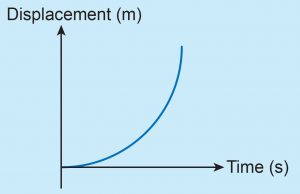# Formative Practice 11.3 – Form 4 Science (KSSM) Chapter 11

Question 1:
Fill in the blanks with the correct answer.
(a) Free fall is the motion of an object due to ________ force only.
(b) The non-free fall motion of an object is affected by _________ resistance.
(c) __________ is the acceleration due to gravitational force acting on an object towards the centre of Earth.

(a) gravitational

(b) air

(c) Gravitational acceleration

Question 2:
The graph below shows the free fall of an object.Explain the graph.